NEET  >  31 Year NEET Previous Year Questions: Waves - 3

# 31 Year NEET Previous Year Questions: Waves - 3

Test Description

## 30 Questions MCQ Test Physics Class 11 | 31 Year NEET Previous Year Questions: Waves - 3

31 Year NEET Previous Year Questions: Waves - 3 for NEET 2023 is part of Physics Class 11 preparation. The 31 Year NEET Previous Year Questions: Waves - 3 questions and answers have been prepared according to the NEET exam syllabus.The 31 Year NEET Previous Year Questions: Waves - 3 MCQs are made for NEET 2023 Exam. Find important definitions, questions, notes, meanings, examples, exercises, MCQs and online tests for 31 Year NEET Previous Year Questions: Waves - 3 below.
Solutions of 31 Year NEET Previous Year Questions: Waves - 3 questions in English are available as part of our Physics Class 11 for NEET & 31 Year NEET Previous Year Questions: Waves - 3 solutions in Hindi for Physics Class 11 course. Download more important topics, notes, lectures and mock test series for NEET Exam by signing up for free. Attempt 31 Year NEET Previous Year Questions: Waves - 3 | 30 questions in 60 minutes | Mock test for NEET preparation | Free important questions MCQ to study Physics Class 11 for NEET Exam | Download free PDF with solutions
 1 Crore+ students have signed up on EduRev. Have you?
31 Year NEET Previous Year Questions: Waves - 3 - Question 1

### Equation of a progressive wave is given by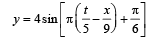Then which of the following is correct? 

Detailed Solution for 31 Year NEET Previous Year Questions: Waves - 3 - Question 1

The standard equation of a progressive wave is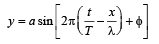The given equation can be written as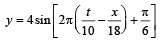∴ a = 4 cm, T = 10 s, λ = 18 cm and φ = π/6

Hence, (b) is correct.

31 Year NEET Previous Year Questions: Waves - 3 - Question 2

### The velocity of sound in any gas depends upon

Detailed Solution for 31 Year NEET Previous Year Questions: Waves - 3 - Question 2

Velocity of sound in any gas depends upon density and elasticity of gas.

31 Year NEET Previous Year Questions: Waves - 3 - Question 3

### If the amplitude of sound is doubled and the frequency is reduced to one fourth, the intensity of sound at the same point will be 

Detailed Solution for 31 Year NEET Previous Year Questions: Waves - 3 - Question 3

Intensity is proportional to (amplitude)2 and also intensity α (frequency)2. Therefore, intensity becomes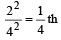31 Year NEET Previous Year Questions: Waves - 3 - Question 4

A 5.5 metre long  string has a mass of 0.035 kg. If the tension in the string is 77N, the speed of a wave on the string is 

Detailed Solution for 31 Year NEET Previous Year Questions: Waves - 3 - Question 4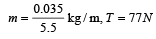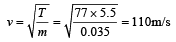31 Year NEET Previous Year Questions: Waves - 3 - Question 5

Velocity of sound waves in air is 330 m/s. For a particular sound wave in air, a path difference of 40 cm is equivalent to phase difference of 1.6π.The frequency of this wave is 

Detailed Solution for 31 Year NEET Previous Year Questions: Waves - 3 - Question 5

From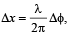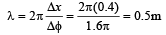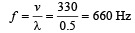31 Year NEET Previous Year Questions: Waves - 3 - Question 6

The transverse wave represented by the equation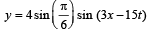has

Detailed Solution for 31 Year NEET Previous Year Questions: Waves - 3 - Question 6

Compare the given equation with standard form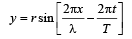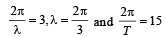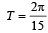Speed of propagation,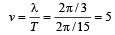31 Year NEET Previous Year Questions: Waves - 3 - Question 7

A closed organ pipe (closed at one end) is excited to support the third overtone. It is found that air in the pipe has 

Detailed Solution for 31 Year NEET Previous Year Questions: Waves - 3 - Question 7

Third overtone has a frequency 7 n, which
means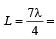three full loops + one half loop, which would make four nodes and four antinodes.

31 Year NEET Previous Year Questions: Waves - 3 - Question 8

Two trains move towards each other with the same speed. The speed of sound is 340 m/s. If the height of the tone of the whistle of one of them heard on the other changes 9/8 times, then the speed of each train should be 

Detailed Solution for 31 Year NEET Previous Year Questions: Waves - 3 - Question 8

Here,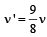Source and observer are moving in opposite direction, therefore, apparent frequency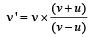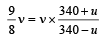⇒ 9 x 340 - 9u = 8 x 340 + 8u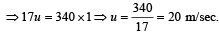31 Year NEET Previous Year Questions: Waves - 3 - Question 9

With the propagation of a longitudinal wave through a material medium, the quantities transmitted in the propagation direction are

Detailed Solution for 31 Year NEET Previous Year Questions: Waves - 3 - Question 9

With the propagation of a longitudinal wave, energy alone is propagated.

31 Year NEET Previous Year Questions: Waves - 3 - Question 10

The frequency of sinusoidal wave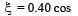[2000 t + 0.80] would be

Detailed Solution for 31 Year NEET Previous Year Questions: Waves - 3 - Question 10

Comparing with the equation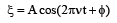We get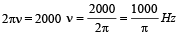31 Year NEET Previous Year Questions: Waves - 3 - Question 11

For production of beats the two sources must have

Detailed Solution for 31 Year NEET Previous Year Questions: Waves - 3 - Question 11

For production of beats different frequencies are essential. The different amplitudes affect the minimum and maximum amplitude of the beats and different phases affect the time of occurrence of minimum and maximum.

31 Year NEET Previous Year Questions: Waves - 3 - Question 12

The temperature at which the speed of sound becomes double as was at 27°C is 

Detailed Solution for 31 Year NEET Previous Year Questions: Waves - 3 - Question 12

We have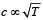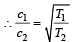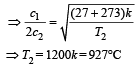31 Year NEET Previous Year Questions: Waves - 3 - Question 13

A stretched string resonates with tuning fork frequency 512 Hz when length of the string is 0.5 m. The length of the string required to vibrate resonantly with a tuning fork of frequency 256 Hz would be 

Detailed Solution for 31 Year NEET Previous Year Questions: Waves - 3 - Question 13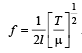When f is halved, the length is doubled.

31 Year NEET Previous Year Questions: Waves - 3 - Question 14

A standing wave is represented by y = Asin (100t) cos (0.01x), where y and A are in millimetre, t in seconds and x is in metre. Velocity of wave is 

Detailed Solution for 31 Year NEET Previous Year Questions: Waves - 3 - Question 14

The wave equation is y = Asin (ωt)cos (kx); c = ω/k = 100/0.01 = 104 m/s.

31 Year NEET Previous Year Questions: Waves - 3 - Question 15

Which of the following equations represent a wave?

Detailed Solution for 31 Year NEET Previous Year Questions: Waves - 3 - Question 15

y = A sin (at – bx + c) represents a wave, where a may correspond to ω and b may correspond to K.

31 Year NEET Previous Year Questions: Waves - 3 - Question 16

A wave of frequency 100 Hz is sent along a string towards a fixed end. When this wave travels back after reflection, a node is formed at a distance of 10 cm from the fixed end of the string. The speeds of incident (and reflected) waves are 

Detailed Solution for 31 Year NEET Previous Year Questions: Waves - 3 - Question 16

As fixed end is a node, therefore, distance between two consecutive nodes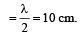λ = 20 cm = 0.2 m

As v = nλ    ∴ v = 100 x 0.2 = 20m/s

31 Year NEET Previous Year Questions: Waves - 3 - Question 17

From a wave equation: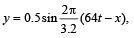the frequency of the wave is 

Detailed Solution for 31 Year NEET Previous Year Questions: Waves - 3 - Question 17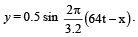Standard equation of the wave is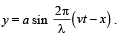Comparing the given equation with the standard equation, we get v = 64 and λ = 3.2.
Therefore, frequency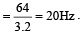31 Year NEET Previous Year Questions: Waves - 3 - Question 18

Two waves are approaching each other with a velocity of 20 m/s and frequency n. The distance between two consecutive nodes is 

Detailed Solution for 31 Year NEET Previous Year Questions: Waves - 3 - Question 18

Distance between two successive nodes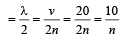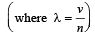31 Year NEET Previous Year Questions: Waves - 3 - Question 19

Two waves are said to be coherent, if they have 

Detailed Solution for 31 Year NEET Previous Year Questions: Waves - 3 - Question 19

The waves, whose frequencies, phases and amplitudes are same at a given time or at a given place in space are known as coherent waves.

31 Year NEET Previous Year Questions: Waves - 3 - Question 20

The speed of a wave in a medium is 760 m/s. If 3600 waves are passing through a point in the medium in 2 min, then their wavelength is 

Detailed Solution for 31 Year NEET Previous Year Questions: Waves - 3 - Question 20

Speed of the wave (v) = 760 m/s; Number of waves = 3600 and time taken (t) = 2 min = 120 sec. Frequecny of waves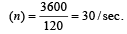.

∴ wavelength of waves  (λ)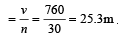[Alt :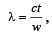where c = velocity of wave t = time in second w = no. of waves]

31 Year NEET Previous Year Questions: Waves - 3 - Question 21

A hospital uses an ultrasonic scanner to locate tumours in a tissue. The operating frequency of the scanner is 4.2 MHz. The speed of sound in a tissue is 1.7 km/s. The wavelength of sound in tissue is close to 

Detailed Solution for 31 Year NEET Previous Year Questions: Waves - 3 - Question 21

Frequency (n) = 4.2 MHz = 4.2 × 106 Hz and speed of sound (v) = 1.7 km/s = 1.7 × 103 m/ s. Wave length of sound in tissue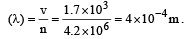31 Year NEET Previous Year Questions: Waves - 3 - Question 22

A source of sound gives 5 beats per second, when sounded with another source of frequency 100/sec. The second harmonic of the source, together with a source of frequency 205/sec gives 5 beats per second. What is the frequency of the source? 

Detailed Solution for 31 Year NEET Previous Year Questions: Waves - 3 - Question 22

Frequency of first source with 5 beats/ sec = 100 Hz and frequency of second source with 5 beats/sec = 205 Hz. The frequency of the first source = 100 ± 5 = 105 or 95 Hz. Therefore, frequency of second harmonic of source = 210 Hz or 190 Hz. As the second harmonic gives 5 beats/ second with the sound of frequency 205 Hz, therefore, frequency of second harmonic source should be 210 Hz or frequency of source = 105 Hz.

31 Year NEET Previous Year Questions: Waves - 3 - Question 23

Two sound waves having a phase difference of 60º have path difference of 

Detailed Solution for 31 Year NEET Previous Year Questions: Waves - 3 - Question 23

Phase difference =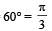Path difference =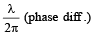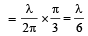31 Year NEET Previous Year Questions: Waves - 3 - Question 24

A star, which is emitting radiation at a wavelength of 5000 Å, is approaching the earth with a velocity of 1.50 × 106 m/s. The change in wavelength of the radiation as received on the earth is 

Detailed Solution for 31 Year NEET Previous Year Questions: Waves - 3 - Question 24

Given : Wavelength (λ) = 5000 Å velocity of star (v) = 1.5 × 106 m/s.
We know that wavelength of the approaching star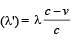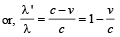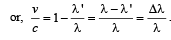Therefore,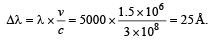[where Δλ = Change in the wavelength]

31 Year NEET Previous Year Questions: Waves - 3 - Question 25

What is the effect of humidity on sound waves when humidity increases? 

Detailed Solution for 31 Year NEET Previous Year Questions: Waves - 3 - Question 25

Velocity of sound =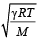When water vapour are present in air, average molecular weight of air decreases and hence velocity increases.

31 Year NEET Previous Year Questions: Waves - 3 - Question 26

The equation of a sound wave is given as: y = 0.0015 sin (62.4 x + 316 t). The wavelength of this wave is 

Detailed Solution for 31 Year NEET Previous Year Questions: Waves - 3 - Question 26

y = 0.0015 sin (62.4x + 316t)

On comparing with y = A sin (ωt + kx)

ω = 316, k = 62.4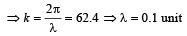31 Year NEET Previous Year Questions: Waves - 3 - Question 27

Two waves of the same frequency and intensity superimpose each other in opposite phases.After the superposition, the intensity and frequency of waves will 

Detailed Solution for 31 Year NEET Previous Year Questions: Waves - 3 - Question 27

We know that interference is said to be constructive at point where resultant intensity is maximum (are in phase) and destructive at points where resultant intensity is minimum or 0 (are in opposite phase). Therefore, after the superposition, frequency and intensity of waves will remain constant.

31 Year NEET Previous Year Questions: Waves - 3 - Question 28

An organ pipe P1 closed at one end vibrating in its first overtone and another pipe P2, open at both ends vibrating in its third overtone are in resonance with a given tuning fork. The ratio of lengths of P1 and P2 respectively are given by

Detailed Solution for 31 Year NEET Previous Year Questions: Waves - 3 - Question 28

We know that the length of pipe closed at one end for first overtone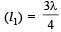and length of the open pipe for third overtone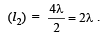Therefore, the ratio of
lengths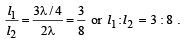31 Year NEET Previous Year Questions: Waves - 3 - Question 29

The speed of a wave in a medium is 960 m/s. If 3600 waves are passing through a point in the medium in 1 min., then the wavelength of the wave is 

Detailed Solution for 31 Year NEET Previous Year Questions: Waves - 3 - Question 29

Given speed of wave (v) = 960m/s

Frequency of wave (f) = 3600/ min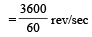= 60 rev per sec.

Wavelength of waves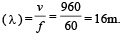[Alt :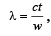where c = velocity of wave  t = time in second w = no. of waves]

31 Year NEET Previous Year Questions: Waves - 3 - Question 30

The equation of a travelling wave is y = 60 cos (180 t – 6x) where y is in microns, t in second and x in metres.The ratio of maximum particle velocity to velocity of wave propagation is 

Detailed Solution for 31 Year NEET Previous Year Questions: Waves - 3 - Question 30

y = 60 cos (180t – 6x)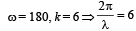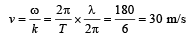Differentiating (1) w.r.t. t,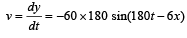vmax = 60 x 180μm/s

= 10800 μm/s = 0.0108 m/s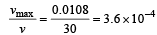## Physics Class 11

127 videos|464 docs|210 tests
 Use Code STAYHOME200 and get INR 200 additional OFF Use Coupon Code
Information about 31 Year NEET Previous Year Questions: Waves - 3 Page
In this test you can find the Exam questions for 31 Year NEET Previous Year Questions: Waves - 3 solved & explained in the simplest way possible. Besides giving Questions and answers for 31 Year NEET Previous Year Questions: Waves - 3, EduRev gives you an ample number of Online tests for practice

## Physics Class 11

127 videos|464 docs|210 tests

### How to Prepare for NEET

Read our guide to prepare for NEET which is created by Toppers & the best Teachers